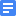Gr4_1-step_(measure first, then 3/4 x 4)Gr4_2-step ((500 - 175) / 6 = n)Gr4_2-step (566 + (566 + 344) = n)Gr4_2-step (700 div 2 div 9 = n)Gr4_2-step (3000 - 425 x 5 = n)Gr4_Multi-step ((20 + 8 + 12) x 5 = n)Gr4_Multi-step (285 + (285 x 3) + (285 + 500) = n)Gr4_Multi-step (1000 - (5 x 84 + 326) = n)Gr4_Multi-step (1200 - (12 x 32 + 12 x 7 + 126) = n)Gr4_Multi-step (2456 - 24 x 4 - 50 x 9 = n)